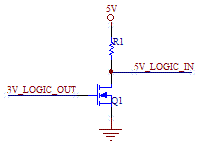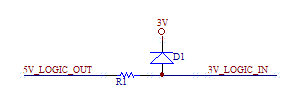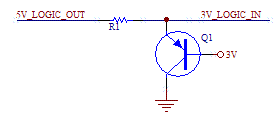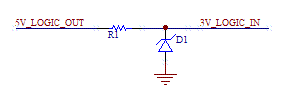# 5V to 3V Level Translator, with Zener clamp.

Interfacing 5V and 3V logic circuits is a common task. 3V logic is preferred to 5V logic because it consumes less power, so most microcontrollers and peripheral devices use the lower voltage logic. However, it is often the case that newer designs using 3V logic will need to interface with the older 5V logic.

Some handy bidirectional commercial ICs such as the ADG3231 from Analog Devices can handle the translation function, but can be cost prohibitive, especially if only a couple of lines need to be translated. These sorts of chips should be used when bi-directionality is needed, or current consumption must be minimized.

Translating 3V to 5V is the easiest task. Usually a 3V output can directly connect to a 5V input. Consult the 5V data sheet to find out the logic switching levels. If more margin is needed a simple transistor circuit can be used as bellow. The FET can be replaced with an NPN bipolar transistor. The disadvantage of this circuit is that it inverts the signal. If a non-inverting signal is a must, then two invertors can be cascaded.Inverting transistor based 3V to 5V Level Translator using MOSFET.

The following circuit uses two diodes to do the translation and is non-inverting.Non-inverting 3V to 5V Level Translator.

Converting from 5V outputs to 3V inputs is a common task. If the 3V device is 5V tolerant, then a straight connection can be made. If the 3V device has input internal clamping diodes then an appropriate series resistor can be used. Otherwise, one of the 3 schemes below can be used. The disadvantage of the diode clamp is that the 3V supply can be pulled higher. The disadvantage can be avoided with the other two approaches, where most of the current is diverted to ground.5V to 3V Level Translator, with diode clamp.5V to 3V Level Translator, with PNP clamp.5V to 3V Level Translator, with Zener clamp.

[Plant Database], [Soil Moisture Sensor] [Water Level Sensor] [Soil Moisture Meter]